Most Affordable JEE | NEET | 8,9,10 Preparation by Kota's Top IITian Doctor Faculties

# Hydrocarbon - JEE Advanced Previous Year Questions with Solutions`
JEE Advanced Previous Year Questions of Chemistry with Solutions are available at eSaral. Practicing JEE Advanced Previous Year Papers Questions of Chemistry will help the JEE aspirants in realizing the question pattern as well as help in analyzing weak & strong areas. Simulator Previous Years JEE Advance Questions
Q. The synthesis of 3-octyne is achieved by adding a bromoalkane into a mixture of sodium amide and an alkyne. The bromoalkane and alkyne respectively are (A) $\mathrm{BrCH}_{2} \mathrm{CH}_{2} \mathrm{CH}_{2} \mathrm{CH}_{2} \mathrm{CH}_{3}$ and $\mathrm{CH}_{3} \mathrm{CH}_{2} \mathrm{C} \equiv \mathrm{CH}$ (B) $\mathrm{BrCH}_{2} \mathrm{CH}_{2} \mathrm{CH}_{3}$ and $\mathrm{CH}_{3} \mathrm{CH}_{2} \mathrm{CH}_{2} \mathrm{C} \equiv \mathrm{CH}$ (C) $\mathrm{BrCH}_{2} \mathrm{CH}_{2} \mathrm{CH}_{2} \mathrm{CH}_{2} \mathrm{CH}_{3}$ and $\mathrm{CH}_{3} \mathrm{C} \equiv \mathrm{CH}$ (D) $\mathrm{BrCH}_{2} \mathrm{CH}_{2} \mathrm{CH}_{2} \mathrm{CH}_{3}$ and $\mathrm{CH}_{3} \mathrm{CH}_{2} \mathrm{C} \equiv \mathrm{CH}$ [IIT-2010]
Ans. (D)
Q. Isomers of hexane, based on their branching, can be divided into three distinct classes as shown in the figure.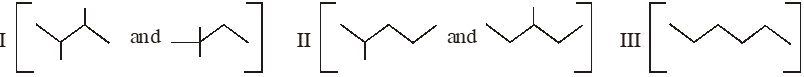The correct order of their boiling point is (A) I > II > III (B) III > II > I (C) II > III > I (D) III > I > II [IIT-2014]
Ans. (B)
Paragraph For Question 3 and 4 Schemes 1 and 2 describe sequential transformation of alkynes M and N. Consider only the major products formed in each step for both the schemes.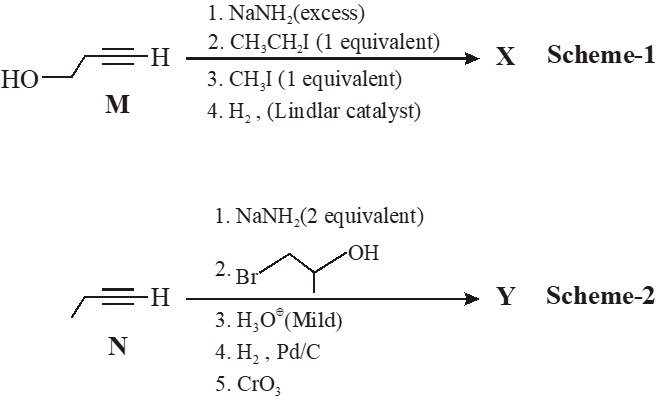Q. The product X is -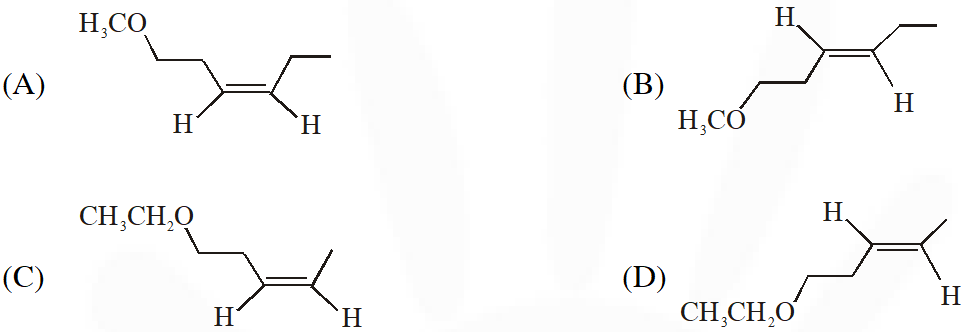[IIT-2014]
Ans. (A)
Q. The correct statement with respect to prodcut Y is – (A) It gives a positive Tollens test and is a functional isomer of X (B) It gives a positive Tollens test and is a geometrical isomer of X (C) It gives a positive Iodoform test and is a functional isomer of X (D) It gives a positive Iodoform test and is a geometrical isomer of X [IIT-2014]
Ans. (C )
Q. Compound(s) that on hydrogenation produce(s) optically inactive compound(s) is (are) –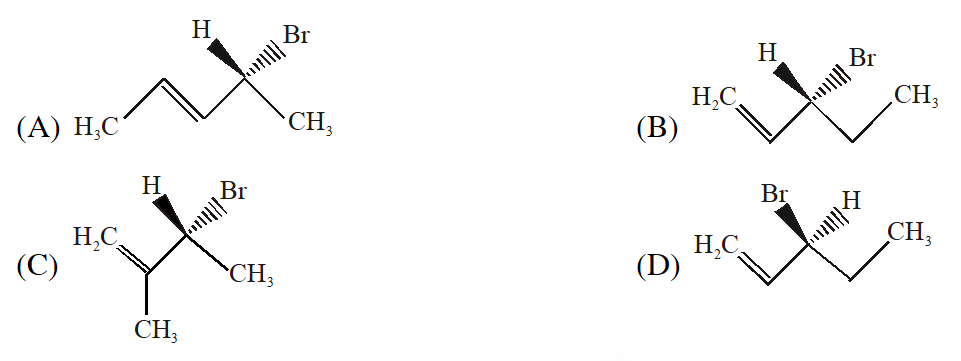[IIT-2015]
Ans. (B,D)
Paragraph For Questions 6 and 7 In the following reaction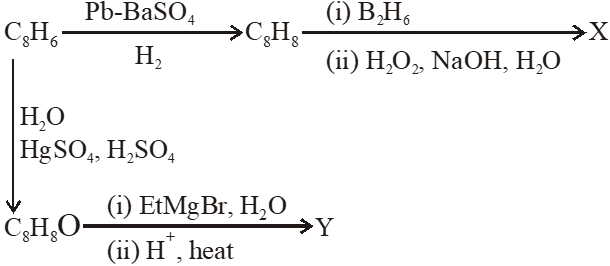Q. Compound X is :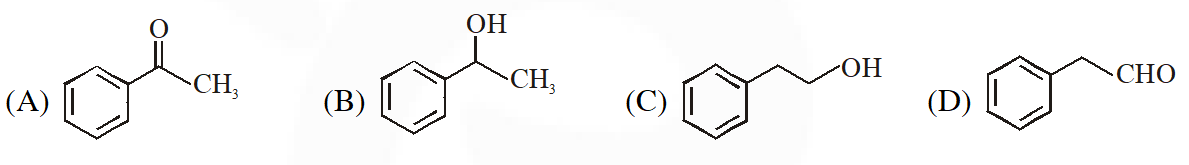[IIT-2015]
Ans. (C)
Q. The major compound Y is :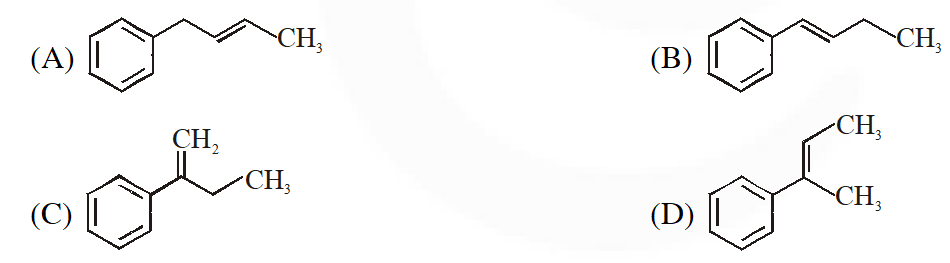[IIT-2015]
Ans. (D)

tejaswi
Aug. 15, 2021, 9:33 a.m.
it would be good if you add total solutions
Prachi
June 27, 2021, 1:02 a.m.
Plz provide aiims questions also
einstein
Dec. 12, 2020, 10:48 p.m.
require many more questions
biligates
Dec. 7, 2020, 9:30 p.m.
I gonna tell that its help full a lot
Abhishekh
May 1, 2020, 8:47 p.m.